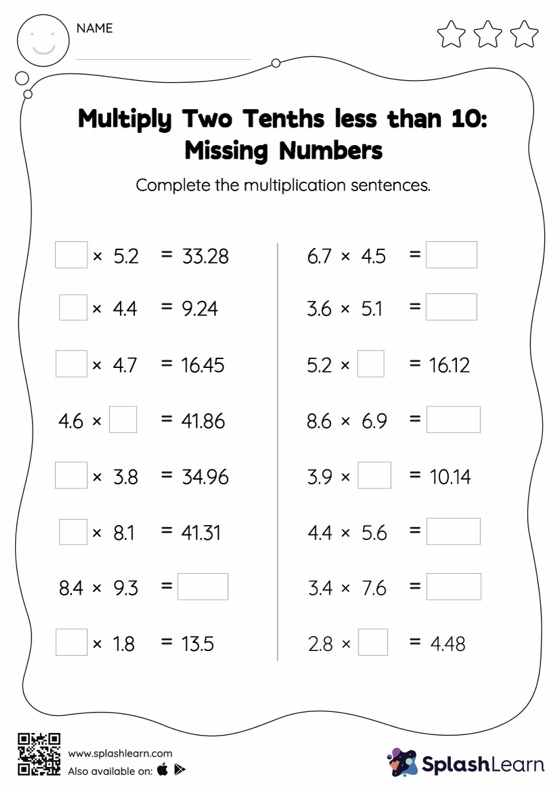# Multiply Two Tenths less than 10: Missing Numbers Worksheet

Home > Multiply Two Tenths less than 10: Missing NumbersWhen a tenth is multiplied by another tenth, the product will have two decimal digits. Students use this understanding to find the missing number as they practice with multiply two tenths less than 10 worksheet. This worksheet is about practicing with the horizontal format in which numbers are written side by side. To develop flexibility with numbers and operations, students need to have enough practice in this format and not just rely on the vertical/column method.